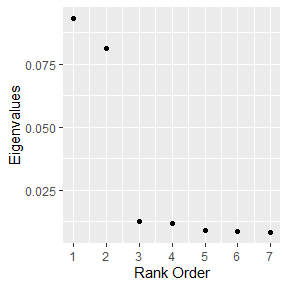# Introduction

In general, dann will struggle as unrelated variables are intermingled with informative variables. To deal with this, sub_dann projects the data onto a unique subspace and then calls dann. sub_dann is able to mitigate the use of noise variables. See section 3 of Discriminate Adaptive Nearest Neighbor Classification for details. Section 4 compares dann and sub_dann to a number of other approaches.

# Arguments

• k - The number of points in the neighborhood. Identical to k in standard k nearest neighbors.
• neighborhood_size - The number of data points used to estimate a good shape for the neighborhood.
• epsilon - Softening parameter. Usually has the least affect on performance.
• weighted - Should the individual between class covariance matrices be weighted? FALSE corresponds to original publication.
• sphere - Type of covariance matrix to calculate.
• numDim - The number of dimensions to project the predictor variables on to.

# Example: Circle Data With Random Variables

In the below example there are 2 related variables and 5 that are unrelated. Lets see how dann, sub_dann, and dann with only the correct features perform. First, lets make a data set to work with.

 library(dann)
library(mlbench)
library(magrittr)
library(dplyr, warn.conflicts = FALSE)
library(ggplot2)

######################
# Circle data with unrelated variables
######################
set.seed(1)
train <- mlbench.circle(500, 2) %>%
tibble::as_tibble()
colnames(train)[1:3] <- c("X1", "X2", "Y")
train <- train %>%
mutate(Y = as.numeric(Y))

train <- train %>%
mutate(
U1 = runif(500, -1, 1),
U2 = runif(500, -1, 1),
U3 = runif(500, -1, 1),
U4 = runif(500, -1, 1),
U5 = runif(500, -1, 1)
)

test <- mlbench.circle(500, 2) %>%
tibble::as_tibble()
colnames(test)[1:3] <- c("X1", "X2", "Y")
test <- test %>%
mutate(Y = as.numeric(Y))

test <- test %>%
mutate(
U1 = runif(500, -1, 1),
U2 = runif(500, -1, 1),
U3 = runif(500, -1, 1),
U4 = runif(500, -1, 1),
U5 = runif(500, -1, 1)
)

As expected, dann is not permanent.

 dannPreds <- dann_df(
formula = Y~X1 + X2 + U1 + U2 + U3 + U4 + U5,
train = train, test = test,
k = 3, neighborhood_size = 50, epsilon = 1, probability = FALSE)
mean(dannPreds == test$Y) ##  0.668 Moving on to sub_dann, the dimension of the subspace should be chosen based on the number of large eigenvalues. The graph suggests 2 (the correct answer).  graph_eigenvalues_df(formula = Y~X1 + X2 + U1 + U2 + U3 + U4 + U5, train = train, neighborhood_size = 50, weighted = FALSE, sphere = "mcd")While continuing to use unrelated variables, sub_dann did much better than dann.  subDannPreds <- sub_dann_df(formula = Y~X1 + X2 + U1 + U2 + U3 + U4 + U5, train = train, test = test, k = 3, neighborhood_size = 50, epsilon = 1, probability = FALSE, weighted = FALSE, sphere = "mcd", numDim = 2) mean(subDannPreds == test$Y)
##  0.882

As an upper bound on performance for this A.I. approach, lets try dann using only the informative variables. Is there much of a difference?

 variableSelectionDann <- dann_df(formula = Y~X1 + X2,
train = train, test = test,
k = 3, neighborhood_size = 50, epsilon = 1, probability = FALSE)

mean(variableSelectionDann == test\$Y)
##  0.944

Using only the related variables produced the best model. Many times, the related variables are unknown. sub_dann was able to produce a model nearly as performant.